# SouthainTemp: MEGR 3171 IOT Project

Ever wanted a temperature sensor that can read the food's temperature? Look no further, this amazing new product is tailored just for you.

BeginnerFull instructions providedOver 1 day250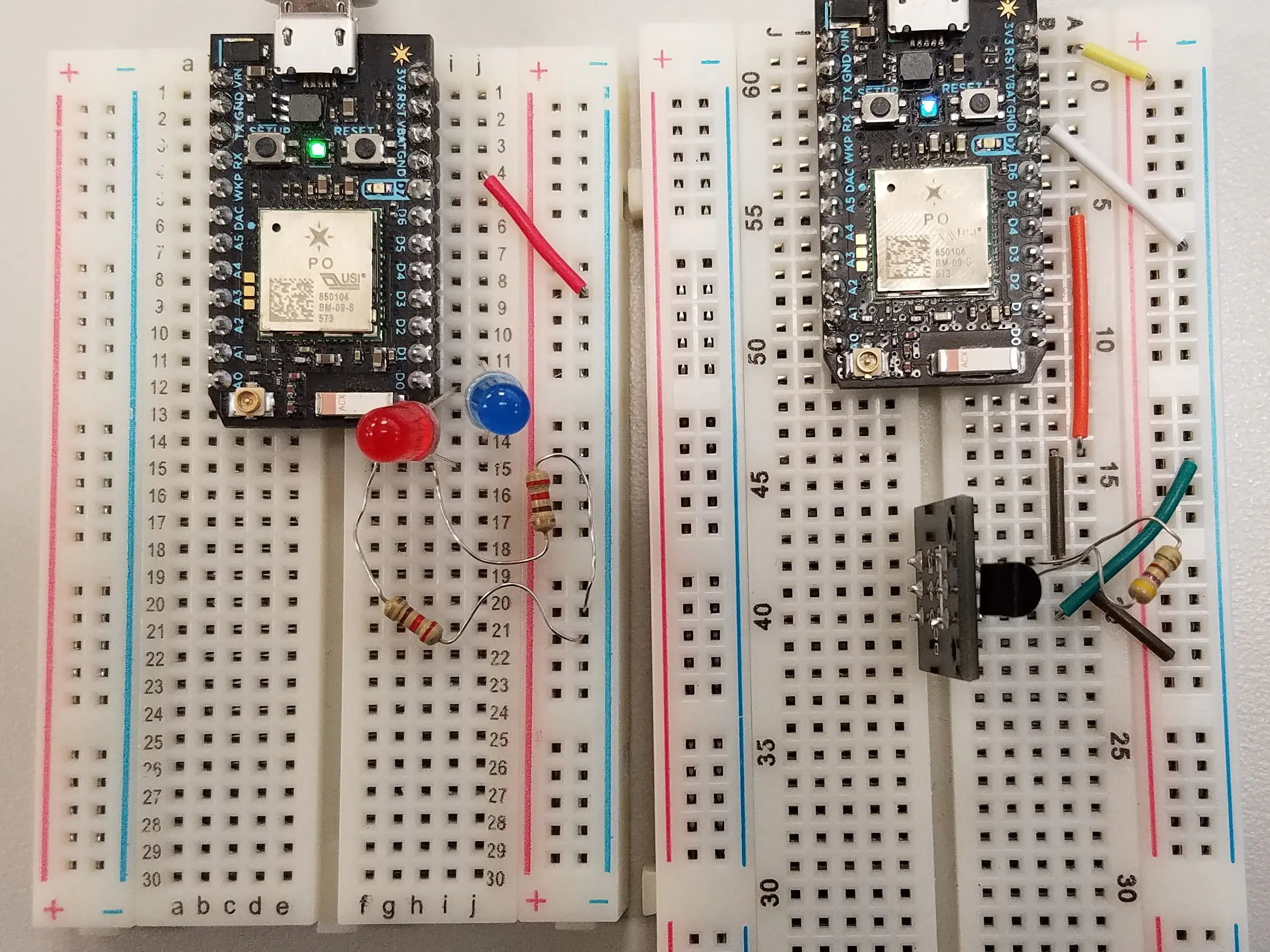## Things used in this project

### Hardware componentsParticle Photon
×2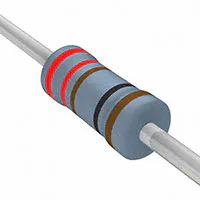Resistor 221 ohm
×2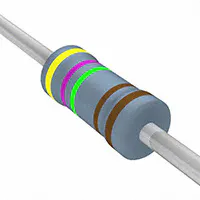Resistor 4.75k ohm
×1LED (generic)
×2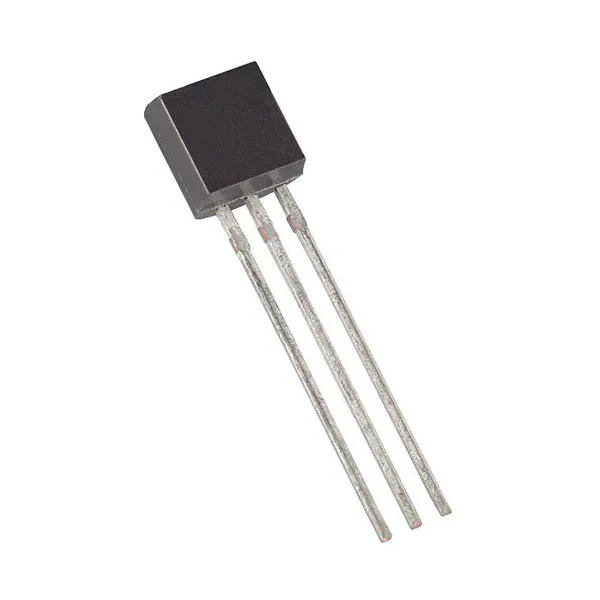Maxim Integrated DS18B20 Programmable Resolution 1-Wire Digital Thermometer
×1

### Software apps and online services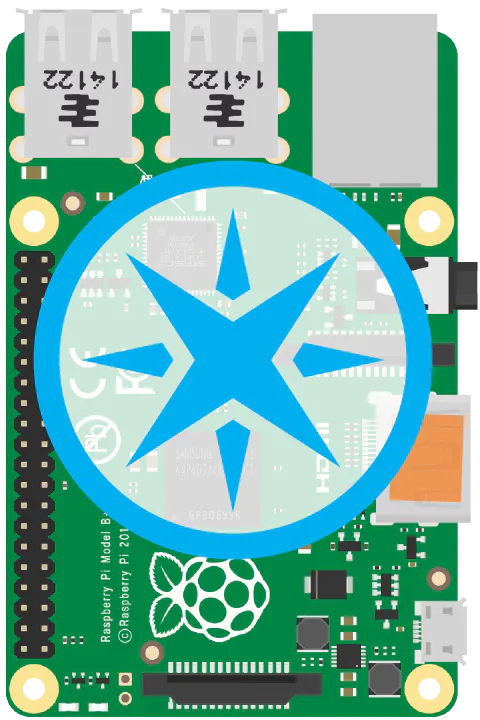Particle Pi

## Schematics

### Sensor

The Sensor Breadboard Diagram for the Project. There are a temperature sensor, DS18B20, and 4.7k ohms resistor attached. Along with those, there are two grounds and two powers wires attached.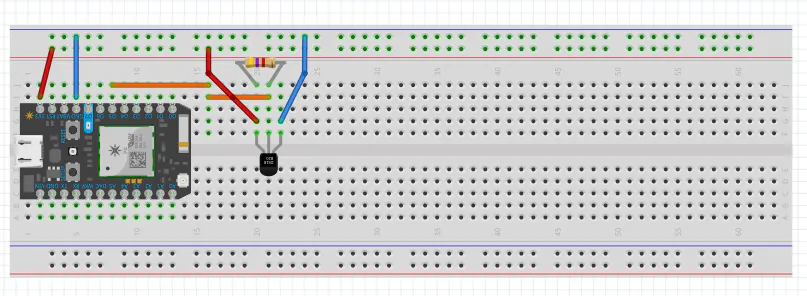### Display

The Display Breadboard Diagram for the Project For the Display, there are two LED displays attached, one red and one blue. Along with them are two 220 ohms resistors. One wire and both resistors go to ground.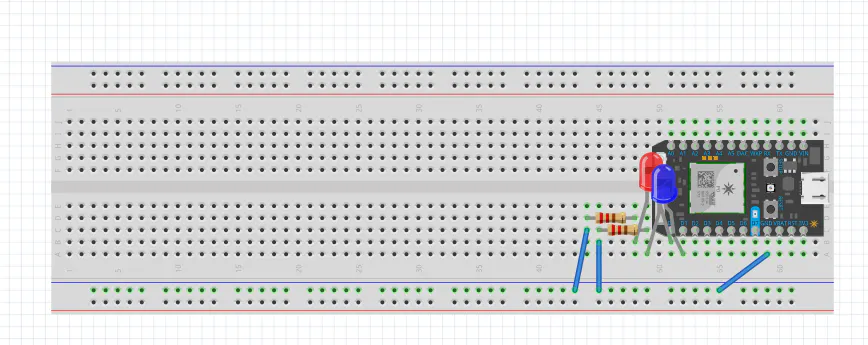## Code

### Display

C/C++
Display code is made to receive the code from Sensor. The code on this end will receive the information and decide whether it is hot or cold and turn the red or blue light respectfully.
```// This #include statement was automatically added by the Particle IDE.
#include <OneWire.h>

// This #include statement was automatically added by the Particle IDE.

OneWire ds = OneWire(D4);  // 1-wire signal on pin D4

//global variables

unsigned long lastUpdate = 0;
float temperature_threshold = 85;
//end global variables

float lastTemp;

void setup() {
Serial.begin(9600);
// Set up 'power' pins, comment out if not used!
//pinMode(D3, OUTPUT);
pinMode(D5, OUTPUT);
//digitalWrite(D3, LOW);
digitalWrite(D5, HIGH);
}

// up to here, it is the same as the address acanner
// we need a few more variables for this example

void loop(void) {
byte i;
byte present = 0;
byte type_s;
byte data;
float celsius, fahrenheit, temperature;

Serial.println("No Sensor Found.");
Serial.println();
ds.reset_search();
delay(250);
return;
}

// The order is changed a bit in this example
// first the returned address is printed

Serial.print("ROM =");
for( i = 0; i < 8; i++) {
Serial.write(' ');
}

// second the CRC is checked, on fail,

Serial.println("CRC is not valid!");
return;
}
Serial.println();

// we have a good address at this point
// what kind of chip do we have?
// we will set a type_s value for known types or just return

// the first ROM byte indicates which chip
case 0x10:
Serial.println("  Chip = DS1820/DS18S20");
type_s = 1;
break;
case 0x28:
Serial.println("  Chip = DS18B20");
type_s = 0;
break;
case 0x22:
Serial.println("  Chip = DS1822");
type_s = 0;
break;
case 0x26:
Serial.println("  Chip = DS2438");
type_s = 2;
break;
default:
Serial.println("Unknown device type.");
return;
}

// this device has temp so let's read it

ds.reset();               // first clear the 1-wire bus
ds.select(addr);          // now select the device we just found
// ds.write(0x44, 1);     // tell it to start a conversion, with parasite power on at the end
ds.write(0x44, 0);        // or start conversion in powered mode (bus finishes low)

// just wait a second while the conversion takes place
// different chips have different conversion times, check the specs, 1 sec is worse case + 250ms
// you could also communicate with other devices if you like but you would need

delay(1000);     // maybe 750ms is enough, maybe not, wait 1 sec for conversion

// we might do a ds.depower() (parasite) here, but the reset will take care of it.

// first make sure current values are in the scratch pad

present = ds.reset();
ds.write(0xB8,0);         // Recall Memory 0
ds.write(0x00,0);         // Recall Memory 0

present = ds.reset();
if (type_s == 2) {
ds.write(0x00,0);       // The DS2438 needs a page# to read
}

// transfer and print the values

Serial.print("  Data = ");
Serial.print(present, HEX);
Serial.print(" ");
for ( i = 0; i < 9; i++) {           // we need 9 bytes
Serial.print(data[i], HEX);
Serial.print(" ");
}
Serial.print(" CRC=");
Serial.print(OneWire::crc8(data, 8), HEX);
Serial.println();

// Convert the data to actual temperature
// because the result is a 16 bit signed integer, it should
// be stored to an "int16_t" type, which is always 16 bits
// even when compiled on a 32 bit processor.
int16_t raw = (data << 8) | data;
if (type_s == 2) raw = (data << 8) | data;
byte cfg = (data & 0x60);

switch (type_s) {
case 1:
raw = raw << 3; // 9 bit resolution default
if (data == 0x10) {
// "count remain" gives full 12 bit resolution
raw = (raw & 0xFFF0) + 12 - data;
}
celsius = (float)raw * 0.0625;
break;
case 0:
// at lower res, the low bits are undefined, so let's zero them
if (cfg == 0x00) raw = raw & ~7;  // 9 bit resolution, 93.75 ms
if (cfg == 0x20) raw = raw & ~3; // 10 bit res, 187.5 ms
if (cfg == 0x40) raw = raw & ~1; // 11 bit res, 375 ms
// default is 12 bit resolution, 750 ms conversion time
celsius = (float)raw * 0.0625;
break;

case 2:
data = (data >> 3) & 0x1f;
if (data > 127) {
celsius = (float)data - ((float)data * .03125);
}else{
celsius = (float)data + ((float)data * .03125);
}
}

// remove random errors
if((((celsius <= 0 && celsius > -1) && lastTemp > 5)) || celsius > 125) {
celsius = lastTemp;
}

fahrenheit = celsius * 1.8 + 32.0;
lastTemp = celsius;
Serial.print("  Temperature = ");
Serial.print(celsius);
Serial.print(" Celsius, ");
Serial.print(fahrenheit);
Serial.println(" Fahrenheit");
temperature = fahrenheit;

if (temperature <= temperature_threshold)
{
Serial.println("fahrenheit <= Threshold");
Particle.publish("IYSCNH", "COLD"); // triggers the light on the second photon to activate by the "Insert Your Specific Code Name Here" cloud.
}
else
{
Serial.println("Attention - Temperature is hot!");
Particle.publish("IYSCNH", "HOT"); // triggers the light on the second photon to activate by the "Insert Your Specific Code Name Here" cloud.
}

// now that we have the readings, we can publish them to the cloud
String temperaturestring = String(fahrenheit); // store temp in "temperature" string
Particle.publish("IYSCNH", temperaturestring, MY_DEVICES); // publish to cloud
delay(2000); // 1 s time delay

}
```

### Sensor

C/C++
The purpose of the sensor to send the capture the data from the DS18B20 temperature sensor and send the data to the Display which will display using the Red or Blue light.
```/* First, let's create our "shorthand" for the pins:
led1 is D0, led2 is D7 */

int led1 = D0;
int led2 = D1;

float temperature;
float temperature_threshold = 55.00;

char temp;

void setup()
{
Serial.begin(9600);
// Here's the pin configuration, same as last time
pinMode(led1, OUTPUT);
pinMode(led2, OUTPUT);
pinMode(D7, OUTPUT);

// For good measure, let's also make sure both LEDs are off when we start:
digitalWrite(led1, LOW);
digitalWrite(led2, LOW);
Particle.subscribe("IYSCNH", ledToggle);

}

void loop()
{
digitalWrite(D7,HIGH);

//display.begin(SSD1306_SWITCHCAPVCC);

}

void myhandler (const char *event, const char *data) {

//    temperature = atof(data);
//    Serial.println(temperature);

}

int ledToggle(const char *event, const char *data) {
/* Particle.functions always take a string as an argument and return an integer.
Since we can pass a string, it means that we can give the program commands on how the function should be used.
In this case, telling the function "on" will turn the LED on and telling it "off" will turn the LED off.
Then, the function returns a value to us to let us know what happened.
In this case, it will return 1 for the LEDs turning on, 0 for the LEDs turning off,
and -1 if we received a totally bogus command that didn't do anything to the LEDs.
*/
String command = String(data);
temperature = atof(data);
Serial.println(temperature);

if (command.equals("HOT")) {
digitalWrite(led1,HIGH);
digitalWrite(led2,LOW);
return 1;
}
if (command.equals("COLD")) {
digitalWrite(led1,LOW);
digitalWrite(led2,HIGH);
return 0;
}
else {
return -1;
}
}
```

## Credits

### Pallove Jain

1 project • 0 followers

### James Souther

1 project • 0 followers
I hope that my circuit will work